## Answers to exercises

##### Exercise 1

We can form a gradient diagram for this function.

 Value of $$x$$ Sign of $$f'(x)$$ Slope of graph $$y=f(x)$$ 1 3 − 0 − 0 + $$\diagdown$$ — $$\diagdown$$ — $$\diagup$$

There is a stationary point of inflexion at $$x=1$$, and a local minimum at $$x=3$$.

##### Exercise 2
Let $$f(x) = x^3-5x^2+3x+2$$. The derivative is $$f'(x) = 3x^2-10x+3 = (3x-1)(x-3)$$. So the stationary points are $$x=\dfrac{1}{3}$$ and $$x=3$$.
• When $$x<\dfrac{1}{3}$$, $$f'(x)>0$$.
• When $$\dfrac{1}{3}<x<3$$, $$f'(x)<0$$.
• When $$x>3$$, $$f'(x)>0$$.
Hence, there is a local maximum at $$x=\dfrac{1}{3}$$, and a local minimum at $$x=3$$.
##### Exercise 3
The inflexion points are $$x=0$$ and $$x=-14$$.
##### Exercise 4
We are given $$f'(x) = x^3(x^2-5) = x^5 -5x^3$$. So the stationary points are $$x=0$$, $$x=\sqrt{5}$$ and $$x= -\sqrt{5}$$. The second derivative is $$f''(x) = 5x^4-15x^2 = 5x^2(x^2 - 3)$$.
• $$f''(0) = 0$$, so we cannot use the second derivative test for $$x=0$$. However, note that $$f'(-1) = 4$$ and $$f'(1) = -4$$. The gradient changes from positive to negative at $$x=0$$. Hence, there is a local maximum at $$x=0$$.
• $$f''(\sqrt{5}) = 50 > 0$$, so there is a local minimum at $$x= \sqrt{5}$$.
• $$f''(-\sqrt{5}) = 50 > 0$$, so there is a local minimum at $$x=- \sqrt{5}$$.
##### Exercise 5

Let $$y = 3x^4-44x^3+ 144x^2$$. Then the first and second derivatives are

\begin{align*} \dfrac{dy}{dx} &= 12x^3-132x^2+288x = 12x(x^2-11x+24) = 12x(x-3)(x-8) \\ \dfrac{d^2y}{dx^2} &= 36x^2-264x+288 = 12(3x^2-22x+24) = 12(3x-4)(x-6). \end{align*}
1. We first find the $$x$$-intercepts: \begin{align*} 3x^4-44x^3+144x^2 &= 0 \\ x^2(3x^2-44x+144) &= 0, \end{align*} which gives $$x=0$$ or $$x=\dfrac{2}{3}(11-\sqrt{13})$$ or $$x=\dfrac{2}{3}(11+\sqrt{13})$$.
2. We have $$\dfrac{dy}{dx}=12x(x-3)(x-8)$$. So $$\dfrac{dy}{dx}=0$$ implies $$x=0$$ or $$x=3$$ or $$x=8$$.
3.  Value of $$x$$ Sign of $$\dfrac{dy}{dx}$$ Slope of graph 0 3 8 − 0 + 0 − 0 + $$\diagdown$$ — $$\diagup$$ — $$\diagdown$$ — $$\diagup$$
4. We use the second derivative test:
• at $$x=0$$, we have $$\dfrac{d^2y}{dx^2} = 288 > 0$$, so there is a local minimum
• at $$x=3$$, we have $$\dfrac{d^2y}{dx^2} = -180 < 0$$, so there is a local maximum
• at $$x=8$$, we have $$\dfrac{d^2y}{dx^2} = 480 > 0$$, so there is a local minimum.
5. As $$x \to \infty$$, $$y \to \infty$$, and as $$x \to -\infty$$, $$y \to \infty$$.
6. $$\dfrac{d^2y}{dx^2} =0$$ implies $$x = \dfrac{4}{3}$$ or $$x=6$$. The second derivative changes from positive to negative at $$x=\dfrac{4}{3}$$, and from negative to positive at $$x=6$$. So there are points of inflexion at $$x=\dfrac{4}{3}$$ and $$x=6$$.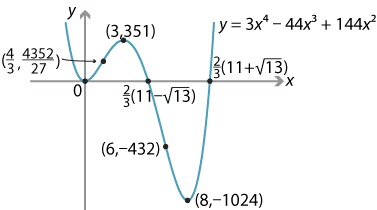##### Exercise 6
Let $$y=4x^3-18x^2+48x-290$$. The first and second derivatives are \begin{align*} \dfrac{dy}{dx} &= 12x^2-36x+48 = 12(x^2-3x+4) \\ \dfrac{d^2y}{dx^2} &= 12(2x-3). \end{align*}
1. The $$y$$-intercept is $$y=-290$$. We now find the $$x$$-intercepts: \begin{align*} 4x^3-18x^2+48x-290 &= 0 \\ 2x^3-9x^2+24x-145 &= 0 \\ (x-5)(2x^2+x+29) &= 0. \end{align*} The quadratic has no real solutions, and so the only $$x$$-intercept is $$x=5$$.
2. $$\dfrac{dy}{dx} = 12(x^2-3x+4)$$. The discriminant is $$12(9-16)<0$$. Hence, $$\dfrac{dy}{dx}>0$$, for all $$x$$, and there are no stationary points.
3. The function is increasing, for all $$x$$.
4. There are no local maxima or minima.
5. As $$x \to \infty$$, $$y \to \infty$$, and as $$x \to -\infty$$, $$y \to -\infty$$.
6. $$\dfrac{d^2y}{dx^2} = 12(2x-3)$$. The second derivative changes from negative to positive at $$x=\dfrac{3}{2}$$. So there is a point of inflexion at $$x=\dfrac{3}{2}$$.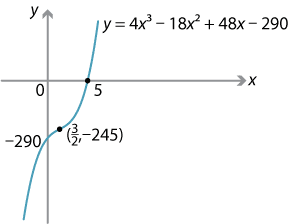##### Exercise 7
Define $$f \colon (0,\infty) \to \mathbb{R}$$ by $$f(x) = x^2\log_e x$$. Then \begin{align*} f'(x) &= 2x\log_e x + x^2 \times \dfrac{1}{x} = 2x\log_e x + x = x(2\log_e x + 1) \\ f''(x) &= 2\log_e x + 2 + 1 = 2\log_e x + 3. \end{align*}
1. For $$x > 0$$, we have $$f(x) = 0 \iff \log_e x = 0 \iff x = 1$$. So the $$x$$-intercept is 1.
2. We have $$f'(x) = x(2\log_e x + 1)$$. So $$f'(x) = 0$$ implies $$x = e^{-\frac{1}{2}}$$. There is a stationary point at $$x = e^{-\frac{1}{2}}$$.
3. We have $f'(x) > 0 \ \iff\ x(2\log_e x + 1) > 0 \ \iff\ 2\log_e x + 1 > 0 \ \iff\ x > e^{-\frac{1}{2}},$ and so $$f'(x) < 0 \iff 0 < x < e^{-\frac{1}{2}}$$.
4. $$f''(e^{-\frac{1}{2}}) = 2 > 0$$. Hence, there is a local minimum at $$x = e^{-\frac{1}{2}}$$.
5. As $$x \to \infty$$, $$f(x) \to \infty$$.
6. We have $$f''(x) = 2\log_e x + 3$$. So $f''(x) > 0 \ \iff\ x > e^{-\frac{3}{2}}, \qquad f''(x) < 0 \ \iff\ 0 < x < e^{-\frac{3}{2}}.$ There is a point of inflexion at $$x=e^{-\frac{3}{2}}$$.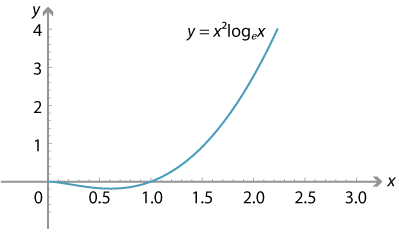A second graph is drawn here to highlight the important points.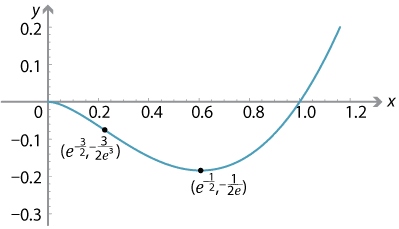##### Exercise 8
Define $$f \colon [-1,2] \to \mathbb{R}$$ by $$f(x) = x^2(x+4)$$. The first and second derivatives are $f'(x) = 3x^2+8x,\qquad f''(x) = 6x+8.$ So $$f'(x) = 0$$ when $$x=0$$ or $$x=-\dfrac{8}{3}$$. The second value is outside the required domain. Since $$f''(0) = 8 > 0$$, there is a local minimum at $$x=0$$, with $$f(0) = 0$$. We now find the value of the function at the endpoints: $$f(-1) = 3$$ and $$f(2) = 24$$.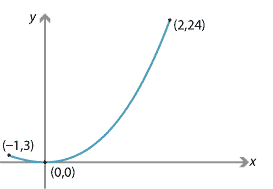The minimum value of the function is 0 and the maximum value is 24.

##### Exercise 9

Let $$x$$ km, $$x$$ km and $$y$$ km be the lengths of fencing of the three sides of the rectangle to be enclosed. Then $$y = 8 - 2x$$.

Let $$A(x)$$ km$$^2$$ be the area of the enclosed land. Then $$A(x) = x(8-2x) = 8x-2x^2$$. The first two derivatives are

$A'(x) = 8 -4x \qquad\text{and}\qquad A''(x) = -4.$

So $$A'(x) = 0$$ implies $$x = 2$$. Since $$A''(2) = -4 < 0$$, there is a local maximum at $$x = 2$$. The rectangle of maximum area has dimensions 2 km $$\times$$ 4 km, and the maximum area is 8 km$$^2$$.

##### Exercise 10
1. The ellipse has equation $$\dfrac{x^2}{16}+\dfrac{y^2}{9} = 1$$.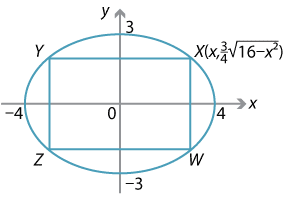Detailed description
Assume the top-right corner of the rectangle is at $$X(x, \dfrac{3}{4}\sqrt{16-x^2})$$. The area $$A$$ of the rectangle $$XYZW$$ is given by $A = XY \times XW = 2x \times \dfrac{3}{2}\sqrt{16-x^2} = 3x\sqrt{16-x^2}.$ We have \begin{align*} \dfrac{dA}{dx} &= 3\Bigl(\sqrt{16-x^2}-\dfrac{x^2}{\sqrt{16-x^2}}\Bigr) \\ &= 3\Bigl(\dfrac{16-x^2-x^2}{\sqrt{16-x^2}}\Bigr) \\ &= 3\Bigl(\dfrac{16-2x^2}{\sqrt{16-x^2}}\Bigr). \end{align*} So $$\dfrac{dA}{dx}=0$$ implies $$x=2\sqrt2$$. Furthermore, we have $\dfrac{d^2A}{dx^2} = \dfrac{6x(-24+x^2)}{(16-x^2)^{\frac{3}{2}}},$ and so $$\dfrac{d^2A}{dx^2} <0$$ when $$x =2\sqrt2$$. The maximum area is $$3\times 2\sqrt 2 \times \sqrt{16-(2\sqrt 2)^2} = 24$$. \item The ellipse has equation $\dfrac{x^2}{a^2} + \dfrac{y^2}{b^2} = 1,$ where $$a,b > 0$$. Assume that the top-right corner of the rectangle is at $$(x,y)$$, where $$0 \leq x \leq a$$ and $$0 \leq y \leq b$$. Let $$A$$ be the area of the rectangle. Then \begin{align*} A &= \dfrac{4b}{a}x\sqrt{a^2-x^2} \\ \dfrac{dA}{dx} &= \dfrac{4b(a^2-2x^2)}{a\sqrt{a^2-x^2}}. \end{align*} So $$\dfrac{dA}{dx} = 0$$ implies $$x = \dfrac{a}{\sqrt{2}}$$. The maximum area is $$2ab$$.
##### Exercise 11

Let the cylinder have radius $$r$$ and height $$h$$. Here $$r>0$$ and $$h>0$$, and they are variables. Let the cone have radius $$R$$ and height $$H$$. They are constants.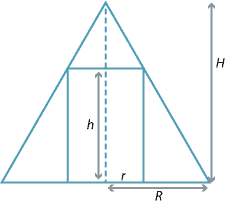The volume $$V$$ of the cylinder is given by $V = \pi r^2 h.$ Using similar triangles, we have \begin{align*} \dfrac{H-h}{r} &= \dfrac{H}{R} \\ HR-hR &= rH \\ h &= H-\dfrac{rH}{R}. \end{align*} Substitute for $$h$$ in the formula for $$V$$: $V = \pi r^2\Bigl(H-\dfrac{rH}{R}\Bigr) = \dfrac{\pi H}{R} \bigl( Rr^2 - r^3 \bigr).$ Differentiate with respect to $$r$$: $\dfrac{dV}{dr} = \dfrac{\pi H}{R} \bigl( 2Rr - 3r^2 \bigr) = \dfrac{\pi H r}{R} \bigl( 2R - 3r \bigr).$ Check for stationary points: $$\dfrac{dV}{dr} = 0$$ implies $$r = \dfrac{2R}{3}$$, since $$r\neq 0$$. The second derivative is $\dfrac{d^2V}{dr^2} = \dfrac{\pi H}{R} \bigl( 2R - 6r \bigr).$

So, when $$r = \dfrac{2R}{3}$$, we have $$\dfrac{d^2V}{dr^2} = -2\pi H < 0$$. A local maximum occurs when $$r = \dfrac{2R}{3}$$. This gives the maximum volume of the cylinder, which is $$V = \dfrac{4\pi R^2H}{27}$$.

##### Exercise 12

The point $$P$$ is moving along the curve $$y = \sqrt{x^3+56}$$. We have

$\dfrac{dy}{dx} = \dfrac{3x^2}{2\sqrt{x^3+56}},$

and so

$\dfrac{dx}{dt} = \dfrac{dx}{dy} \times \dfrac{dy}{dt} = \dfrac{2\sqrt{x^3+56}}{3x^2} \times \dfrac{dy}{dt}.$

When $$x=2$$, we are given that $$\dfrac{dy}{dt} = 2$$, and so

$\dfrac{dx}{dt} = \dfrac{2\sqrt{2^3+56}}{3 \times 2^2} \times 2 = \dfrac{8}{3}.$
##### Exercise 13

Assume the meteor is a sphere of radius $$r$$. Its surface area is $$S = 4\pi r^2$$, and its volume is $$V = \dfrac{4}{3} \pi r^3$$. The volume is decreasing at a rate proportional to the surface area. That is,

$\dfrac{dV}{dt} = -kS = -4\pi k r^2,$

for some positive constant $$k$$. Using the chain rule, we have

$\dfrac{dr}{dt} = \dfrac{dr}{dV} \times \dfrac{dV}{dt} = \dfrac{1}{4\pi r^2} \times -4\pi k r^2 = -k.$

So the radius is decreasing at a constant rate.